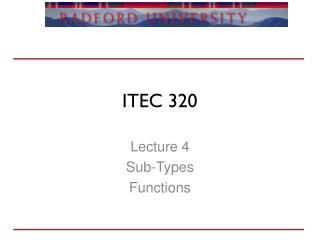DownloadDownload PresentationITEC 320

# ITEC 320

Télécharger la présentation## ITEC 320

- - - - - - - - - - - - - - - - - - - - - - - - - - - E N D - - - - - - - - - - - - - - - - - - - - - - - - - - -
##### Presentation Transcript

1. ITEC 320 Lecture 4 Sub-Types Functions

2. Review • Types • Homework • Questions?

3. Outline • Subtypes / Enumerated types • Procedures • Functions • Problems

4. Compiler tasks • Compile time • What errors are caught? • Run-time • What errors are caught

5. New types • Example type Temperature is range 32 .. 212; type Pressure is range 0 .. 500; t: Temperature; p: Pressure; t := 100; -- valid p := t; -- compile error

6. Enumerated types • Map an int to a word procedure enum_demo is type Color is (red, blue, green); c: color := green; begin if c = blue then -- do something end if; c:=0; --What happens here? end enum_demo;

7. Modular Types • What do you think this is used for type ClockHourType is mod 12; startTime: ClockHourType := 10; breakTime: ClockHourType := startTime + 2; -- 0 endTime: ClockHourType := startTime + 4; -- 2

8. Floating point • Example type Money is delta 0.01 digits 15; cent: Money := 0.01; dollar: Money := 0.0; for i in 1 .. 100 loop dollar := dollar + cent; end loop; put(float(dollar)); -- 1.00

9. In-class • Let's write a program in groups: Read a low and a high value to define a range, and then read a sequence of numbers, until eof, and count the number of numbers below, in, and above the range. Calculate the percent in each group. Assume the endpoints of the range are in the range. Output, nicely formatted, the counts and percents.

10. Functions • Similar to java methods with a non-void return type • Must have a return type • Must be defined before use • Cannot modify parameters

11. Syntax function sumArray(a: Int_Array) return integer is s: Integer := 0; -- holds the sum begin for i in a'range loop s := s + a(i); end loop; return s; end sumArray;

12. Procedures • No return type allowed • Parameters can be changed • In (default) • Out (allows passing back to the caller) • In Out (pass in and out) • What about the get procedure’s parameter? • Structure charts help

13. Syntax with ada.text_io; use ada.text_io; with ada.integer_text_io; use ada.integer_text_io; procedure inout is procedure vars(a: in Integer; b: in out Integer; c: out Integer) is begin b:= b+a; c:= b+3; end vars; x: Integer; y: Integer; z: Integer; begin x:=2; y:=3; vars(x,y,z); put(x'img & "|" & y'img & "|" & z'img); end inout;

14. Issues • Assigning to an in parameter • Using an out parameter’s values in the procedure (by default it can be anything) • Forgetting to assign a value to an out parameter • Can’t fool variables • I.e. passing an in mode variable to another procedure where it is an out mode parameter

15. Problems • Write a function/procedure that returns whether or not a String is a palindrome

16. Problems • Given n of 1 or more, return the factorial of n, which is n * (n-1) * (n-2) ... 1. Compute the result recursively (without loops).

17. Summary • Functions • Procedures